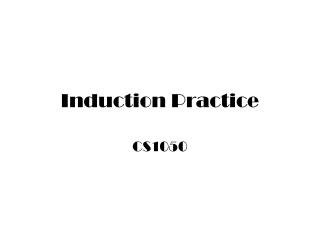Download PresentationInduction Practice

# Induction Practice - PowerPoint PPT PresentationDownload Presentation## Induction Practice

- - - - - - - - - - - - - - - - - - - - - - - - - - - E N D - - - - - - - - - - - - - - - - - - - - - - - - - - -
##### Presentation Transcript

1. Induction Practice CS1050

2. Prove thatwhenever n is a positive integer. Proof: Basis Case: Let n = 1, then

3. Prove thatwhenever n is a positive integer. Inductive Case: Assume that the expression is true for n, i.e., that Then we must show that:

4. If S is a finite set with n elements, then S has 2n subsets (1of 2) We will do a proof by induction. Basis Step: Assume that the set is empty, i.e., that it has 0 elements, then it has 20 = 1 subsets, which is Ø. Inductive Step: Assume that a set with n elements has 2n subsets. Then we must show that this implies that a set with n+1 elements has 2n+1 subsets.

5. (2 of 2) Let S be the set with n elements and T be the set with n+1 elements. Let a be the element that is added to S to get T. So T = S{a} or S = T-{a}. For every subset, X, of S there must be exactly two distinct subsets of T: X and X{a}. 2*2n = 2n+1

6. Let p(n) be the proposition that there exists t,e  N such that 3t + 8e = n. Prove n  14N, p(n) is true. We will do a proof by induction. Basis Step: Prove p(14) Let t = 2 and e =1, then 3(2) + 8(1) = 14. Inductive Step: Assume p(k) is true. Thus there exist t,e N such that 3t+8e = k. We need to show that there exist u,f N such that 3u + 8f = k+1. There are two cases e = 0 (there are no 8’s) or e 1 (there is at least one 8).

7. Case 1: e = 0 Since k  14 and e = 0, then t  5. Let u = t-5 and f = 2. Since t  5, u  N and clearly f  N. Now 3u + 8f = 3(t-5) + 8(2) = 3t+1 = k + 1. (Effectively, take out three five’s and replace with 2 eight’s.)

8. Case 2: e  1 Let u = t+3 and f = e-1. Since t,e  N and e  1, u,f  N. Now 3u + 8f = 3(t+3) + 8(e-1) = 3t + 8e + 1 = k+1. (Effectively what we have done is remove one 8 and replace it with 3 threes to get a net increase of 1)

9. Generalization of De Morgan’s Law = n  2 (pq)(p  q) De Morgan’s Laws (pq)(p  q)

10. Generalization of De Morgan’s Law = n  2 (pq)(p  q) De Morgan’s Laws (pq)(p  q)